Alias in the Physics package...

Dear all,

I am trying to use another notation for the inert differential operators in the Physics package (using alias), I am not getting the correct result. Does anyone know how I can solve this issue? Many thanks!How to solve the differential equation with piecew...

I want to solve a differential equation with piecewise function, but I face an error. I am wondering how to handle it. Thanks

t:=1

x := t -> piecewise(0 <= t and t < t, -60*t + 100, t <= t and t <= 10, 1.645*sqrt(480*t))

dsolve({diff(p(t), t) = 2*h*x(t), p(T) = 0}, p(t))

and the error is :

"Error, (in dsolve) found the following equations not depending on the unknowns of the input system: {pT = 0}"(1)(2)Animation of an iron head pump...

I am trying to anime a pump but itn't working. I need help. Thank you. restart; with(plots); with(plottools); unprotect(D); alpha := arctan(-58/62.5); solve({k*Pi/100 = alpha}, {k}); beta := arctan(19/84); solve({k*Pi/100 = beta}, {k}); ang1 := arctan(-68/84); evalf(solve({k = ang1}, {k})); ang2 := arctan(55/84); evalf(solve({k = ang2}, {k})); #angular head travel Head := proc(k) local r, poly, k1, tC, tD, tE, DikC, DikD, DikE; global C, D, E; r := 84; C := [-55, 17]; D := [0, 0]; E := [84, 0]; poly := [[-60, 24], [63, -17], [60, -55.5], seq([r*cos(1/100*k*Pi), r*sin(1/100*k*Pi)], k1 = -24 .. 7), [82, 18], [78, 20], [64, -3], [-46.5, 35]]; tC := textplot([C[], "C"], align = {above, right}, font = [Times, bold, 18]); tD := textplot([D[], "D"], align = {above, left}, font = [Times, bold, 18]); tE := textplot([E[], "E"], align = {above, left}, font = [Times, bold, 18]); DikC := disk(C, 1, color = black); DikD := disk(D, 1, color = black); DikE := disk(E, 1, color = black); if 0

does Maple have method to factor on specific symbo...

I am looking for a robust way to factor an expression (if applicable) to become    x^n*(rest)  as we do it by hand.

edit The input will only be of type `+` and I am looking for a way extract a common factor to convert the input to term^n*(rest) where term is the common factor to pull out.

For example, given  x^2*Y+x and the symbol is given as x  the result will be x*(x*Y+1) and if the input is Y^2*x^3-x^3 then the output is x^3*(Y^2-1) and if there is no common factor x to pull out from all the terms, the output will be the same as the input.

I tried many commands and options, but can't find one method that works all the time for all cases.

For example for   x^2*Y+x  the command factor(x^2*Y+x) gives (Y - 1)*(Y + 1)*x^3 which is not what I want. There is no option to factor to give the name to factor on. And I did not know how to use the last argument of function to do that.

But here simplify(x^2*Y+x) happened to work on this and gives (Y^2 - 1)*x^3 but simplify does not work on first example. simplify(x^2*Y+x) returns the same expression back. So simplify is not reliable to use.

I tried collect, with options factor and simplify. Again, they work on one examples but not others.

collect(x^2*Y+x,x); does not do it. But collect(Y^2*x^3-x^3,x); works and returns (Y^2 - 1)*x^3 which is what I want.

The problem is that I do not know what the expression looks like. I just know the name and want to find if there is a common to any power that can be pulled out to rewite the expression as x^n*(rest) where is an integer or rational number depending.

This seems like a simple problem. But can't find a Maple command for.   I could ofcourse program it by brute force. Go over each term in the expression, check if each term has a free to any power in it multiplied by something else, then collect all these x^n term in a list. At end find the which is raised to lowest power, and then divide the whole expression by it.

Here is another way I can also try:  Use factor and also collect and also simplify. One at a time. Each time I check if the result is of type `*` but not a division! (check that denom is 1). If so, Then check if result has two operands only. If so, check if op(1,result) is for form x^anything. If so, then one of these cases worked.  Need to try this now to see if it will work on all cases I have.

Is there a better way to do this in Maple? It has to work on all expresions f(x) without knowing what the expression looks like.

update

I've updated the test cases and included all algorithms given to compare. It is hard in Maple to make a nice table to present results and keep math formatting below.

restart;
makegrid := proc(M::Matrix)#https://www.mapleprimes.com/questions/202902-How-To-Create-Table-Like-Output-For
uses DocumentTools:-Layout;
local i,j,m,n,wks;
m,n := op(1,M);
wks := Worksheet(Table(alignment=center,width=20,
seq(Column(),j=1..n),
seq(Row(seq(Cell(Textfield(sprintf("%a",M[i,j]))),
j=1..n)),i=1..m)));
DocumentTools:-InsertContent(wks);
end proc:

acer_V1_common_factor := proc(x::algebraic, ee::algebraic)
local p, d := gcd(ee, x^frontend(degree,[ee,x]),'p');
d * p;
end proc:

acer_V2_common_factor := proc(x, ee) local d, t;
if ee::`+` then
t := max(map(proc(u) local r:=frontend(degree,[u,x]);
`if`(r::numeric,r,0); end proc,[op(ee)]));
d := gcd(numer(ee),x^t);
d*map(u->u/d,ee);
else ee; end if;
end proc:

dharr_common_factor:=proc(x,z)
local xn:=x^ldegree(collect(z,x),x);
if rem(z,xn,x)=0 then xn*quo(z,xn,x) else z end if;
end proc:

me_common_factor:=proc(term,expr)
local tmp;
local T1;

local update_T1:=proc()
T1:= hastype(op(1,tmp),identical(term)^anything) or hastype(op(1,tmp),identical(term));
if not T1 then
T1:= hastype(op(2,tmp),identical(term)^anything) or hastype(op(2,tmp),identical(term));
fi;
end proc;

if type(expr,`*`) or not has(expr,term) then
return expr;
fi;

tmp := collect(expr,term);
if type(tmp,`*`) and evalb(denom(tmp)=1) and evalb(nops(tmp)=2) then
update_T1();
if T1 then
return tmp;
fi;
fi;

tmp :=factor(expr);
if type(tmp,`*`) and evalb(denom(tmp)=1) and evalb(nops(tmp)=2) then
update_T1();
if T1 then
return tmp;
fi;
fi;

tmp := simplify(expr);
if type(tmp,`*`) and evalb(denom(tmp)=1) and evalb(nops(tmp)=2) then
update_T1();
if T1 then
return tmp;
fi;
fi;

return expr;
end proc:
############################

test_data:=[[x,x^2*Y+x],
[x,Y^2*x^3-x^3],
[x,x],
[x,x+2*x^2],
[x,x^4*diff(y(x),x)+x^7],
[x,x^4*diff(y(x),x)+x^7-sin(x)],
[y(x),y(x)^4*diff(y(x),x\$2)^2*diff(y(x),x)+y(x)^2*diff(y(x),x)+y(x)],
[y(x),y(x)^4*diff(y(x),x\$2)^2+y(x)^2*diff(y(x),x)+y(x)^9],
[x,x^4*y^2+x^2*y^2],
[y(x),y(x)^4*diff(y(x),x)^2+y(x)^2*diff(y(x),x)^2],
[diff(y(x),x),y(x)^4*diff(y(x),x)^2+y(x)^2*diff(y(x),x)^2],
[diff(y(x),x),y(x)*diff(y(x),x\$2)^2*diff(y(x),x)*sin(x)+diff(y(x),x)^3],
[y(x),diff(y(x),x)-(1+x^(1/2))/(1+y(x)^(1/2))],
[y(x),diff(y(x),x) -(x-1)*y(x)^5/x^2/(-y(x)+2*y(x)^3)],
[y(x),3*y(x)+diff(y(x),x) - 2*x/exp(3*x)],
[x,3*x^2*y^3+7*x/y],
[y,A-(1+x)/(1+y^(1/2))]
]:
RESULT:=Matrix(nops(test_data),6);
for N,item in test_data do
term:=item;
expr:=item;
RESULT[N,1]:=term; RESULT[N,2]:=expr;
try
result:=acer_V1_common_factor(term,expr);
if type(result,`*`) and denom(result)<>1 then
RESULT[N,3]:=expr;#bypass, not correct output
else
RESULT[N,3]:=result;#accept
fi;
catch:
RESULT[N,3]:=expr;#reject
end try;

try
result:=acer_V2_common_factor(term,expr);
if type(result,`*`) and denom(result)<>1 then
RESULT[N,4]:=expr;#bypass, not correct output
else
RESULT[N,4]:=result;#accept
fi;
catch:
RESULT[N,4]:=expr;#reject
end try;

try
result:=dharr_common_factor(term,expr);
if type(result,`*`) and denom(result)<>1 then
RESULT[N,5]:=expr;#bypass, not correct output
else
RESULT[N,5]:=result;#accept
fi;
catch:
RESULT[N,5]:=expr;#reject
end try;

try
result:=me_common_factor(term,expr);
if type(result,`*`) and denom(result)<>1 then
RESULT[N,6]:=expr;#bypass, not correct output
else
RESULT[N,6]:=result;#accept
fi;
catch:
RESULT[N,6]:=expr;#reject
end try;

od:

RESULT

how_to_do_special_factor.mw

Mapleprime will not let let insert content for some reason. Here is the output as screen shot but it is hard to read. But it is in the above worksheet.Maple 2021 Linux installation failed! "Missing hos...

Hello everyone,

I purchased Maple 2021 student version. I am using a Linux Ubuntu 20.04 machine. The machine is my personal computer, so there is no user access privilege restriction. I installed Maple using the GUI, and at the activation step, I get an error that says "Missing host ID for license server". I remember an option for servers or personal PC during download. I am confident that I chose the personal PC option.

Kind regards, and I look forward to hearing from you.

Taking a list of numeric values L...

"Taking a list of numeric values L, and producing a list of lists [p,f(p)] for every p in L , is not complicated. In fact it is a quite straightforward and rather basic example of Maple programming"

This sentence i don't understand yet, so i must see a example?

Creating dataplots...

Hey guys,

How can I create a bar chart or a column chart, with specific x values under the bars.

Would be great If someone could help me.

Thank you;)

How to put a function in the Given ordinary Differ...

Help.mw

How to put a function in the ODE. I have attached below work here.

Thank you

What hyperbolic transformation performs this works...

The worksheet below contains the desired hyperbolic action and asks for the specific transformation that performs it.

Hyperbolic_Transformation.mw

I have corrected the above worksheet so that the Poincare disk is now centred at [0,0].

I hope this helps you answer my question

Cyclic polynomials....

Is there some basis containing polynomials of the form x^p*x^q+x^p*x^q+x^p*x^q, (p,q posint)?
Are there known relationships between these?

Note: these are not the symmetric polynomials.

How to factor out specific parts of expression...

Kitonum had provided us the basis for a solution to this question.  I created a proc to make it versatile.
The problem was how to force Maple to display the RHS of :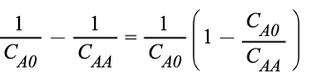Here is the proc(I've condensed the variables)

extract := proc(a, expr)
local f:
f := a:
``(f)*expand(expr/f);
end proc:

eq := 1/cao - 1/caa:
extract(1/cao, eq);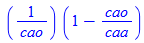Now suppose we had an trigonometric expresssion and we extract cos(x) out of it

expr := cos(x) - sin(x)/(x^2*y) + 3*x*y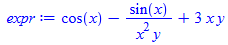bb := extract(cos(x), expr)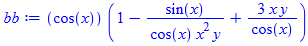ok, that's good, now we would like to keep the order but simplify the middle term sin/cos to tan.  The best way about it is to use algsubs and manually substitute the relation with the option exact.  I don't Maple can achieve this without the manual help.

algsubs(sin(x)/cos(x) = tan(x), bb, exact)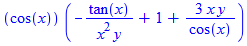Now we're at the mercy of Maple here.  Maple always likes to throw negative terms out front.  The premise of Maple is to show it as it would appear on paper.  I mentioned exact and it shouldn't have changed the order but it did.  So maybe if we use sort we can right it.

sort(%)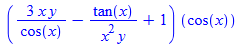Ok, good but now Maple has thrown the single term multiplier at the end.

Can anyone get maple to put the cos(x) term out front?

documentation is not updated in some places....

help under object it says  (in Maple 2021)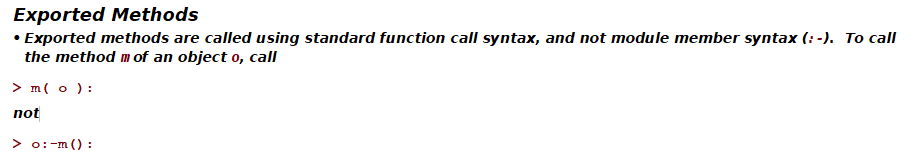The above has not been true for almost a year. Since in Maple 2021 one can do o:-m()

person_class :=module()
option object;
export m:=proc(_self,\$)
print("you called me");
end proc;
end module;

o:=Object(person_class);
o:-m()

person_class := Object<<1758956556512>>
o := Object<<1758955674784>>
"you called me"

I hope this gets updated in Version 2022,  with examples also. The notation o:-method() is hidden in help and could not even find it, but it is meantioned in some pdf file which I lost track of at this moment.

On another help page titled Overview of Object Methods it briefly mentions alternative call but the link that is supposed to explain it does not work. (clicking on it, produces nothing. Stays on same page). I guess no one tried this link before shipping Maple.

Is this link somewhere to access directly in the help page?maple's factor option....

How can factorise a polynomial with complex roots, without showing their numerical values of the roots, but only their symbolical value.

For example I have:

factor(x^4 - x^2 + x - 1)

and it shows me the following factorisation: (x-1)(x^3+x^2+1) but I want to also factorise x^3+x^2+1 but the roots should be displayed symbolically without numerical values of these roots. Then how to implement this task?

In the book I am reading it's stated to use factor(x^4-x^2+x-1, I), but it doesn't seem to be working.

Minimum positive integer solution of system...

Hi.

I have a question, if it is possible please guide me. I tried for this problem but I failed.

I have a set of linear equations that I want to find the "minimum" "positive" "integer" solution to it. This system may contain free parameters in some cases that they should be set an integer in such a way that they yield our goal namely "Minimum positive solution".

I used isolve but it does not work for this case.

Indeed I especially want that maple use isolve it and then search that if there are "freevars" (in the solution: produced by maple when we use isolve code) or not, if yes then set number such that all solutions be the minimum positive integer set that we can have, indeed, the "freevars" should not set manually, but by the Maple. In the case that there is no "freevar", then maple should give me the minimum positive integer that we can have.

Note that the result must be a unique set of solutions.

For example, one of such systems that I am concerning is as follows which contains freevar in solution when we use isolve.

sys := [a = 2*a, a = a, 4*a+2*a = a+2*a, 2*a+2*a = 2*a, a = a+a, 2*a-a = 2*a]

Divergence or convergence?...

Hello everybody.

I have a short question. I have an expression related to the calculation of the Gaussian curvature

-24.*y^6*(y-1.)^7*(y+1.)^7*(4.+6.*y^8-22.*y^6+30.*y^4-18.*y^2)/(4.*y^18-28.*y^16+84.*y^14+4.-136.*y^12+116.*y^10-24.*y^8-52.*y^6+56.*y^4-24.*y^2)^2

that is diverged for y=1 as (y-1)^(-2).

At the same time substitution of something like y:=1.00001 gives the value almost zero.

What is the trick?

﻿